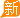• 现代 >
• 北京现代北京现代ix35领动 插电混动## 探秘行业标杆 走进“北京现代PDI”

2014年12月24日 13:22 来源：www.chextx.com 超过：19978次关注

前段时间“PDI”一度成为汽车行业热词，不少消费者在购买汽车时莫名的告知还有一项PDI费用，而在网上随便一搜“汽车PDI”相关内容也数不胜数。汽车PDI究竟是什么？它在汽车生产和销售过程中又起到什么作用？很多消费者包括行业人士对PDI的概念也是一头雾水。近日，笔者就走进了有着汽车行业最大PDI部门的北京现代一探究竟。

说起PDI，可能很多人都会感到一些陌生。其实PDI就是新车售前检测，是Pre Delivery Inspection的英文缩写，这也是汽车销售前的最后一道工序。PDI是在车辆生产过程中质量控制（QC）检验的基础上，从客户的角度，通过检查/维修作业来解决质量问题,确认无问题后再出库。因为新车从生产厂商到达经销商处要经历上千公里的运输和长时间的停放，为了向顾客保证新车的安全性和原厂性能，PDI检查也就变得十分重要。而且越是高档车辆，其电子自动化程度越高，PDI环节所需要检查的项目也就越多。

北京现代的PDI的发展可谓既前瞻又快速。早在2002年成立之初，北京现代就设置了PDI部门，虽然规模不大，但此举在当时的汽车市场中具有相当的前瞻性。而伴随年产销过百万的发展速度，北京现代PDI部门也进入发展的快车道，目前PDI员工近600人，车间占地面积也从2700平米迅速扩大到16500平米。与此同时，先进的洗车房设备和检测线，装备齐全的车辆修复车间，让北京现代的PDI环节变得更加有序而高效。

北京现代如此看重PDI环节，这与其“品质经营”的理念不无关系。北京现代一直坚持将品质理念全面贯彻到设计、采购、生产、销售、售后等各个环节，作为产品质量的最后“把关人”，PDI环节关乎消费者的评价、老百姓的口碑，自然是重中之重。为此，北京现代在PDI的规范化管理、市场调查等方面下了十足的功夫，希望以此来不断的提升产品竞争力及品牌影响力。

首先，是细致入微的新车检查检测。北京现代的PDI检测环节包括车辆清洁、内外观检查、发动机检查、漆面检查、简单维修、法规标签粘贴（燃料标签、惠民标签、CCC 标签）、车衣/车膜粘贴  品质问题维修、投入随车用品等环节。相关数据显示，北京现代PDI检测中，每辆准备出厂的新车平均需要检查260多项内容。

其次，在规范化管理方面，面对如此多的工序和高效的要求，北京现代的PDI 部门制定并完善了一系列的质量标准化管理体系。例如在PDI检查的过程中，相关规范需要细致到什么角度，什么距离，都有着十分明确的说明。这一方面提高了作业人员的专业化水平和工作效率，另一方面，有了这些标准，在PDI检查中，可以进一步保证品质的稳定性，保证将高品质的车辆交付到客户手中。

同时，北京现代的PDI不仅对内要做到规范，对外也要做到充分的了解，市场调研就显得尤为重要。对于汽车企业来说，消费者、客户的满意才是衡量产品的准绳，PDI必须坚持站在客户的角度、不断了解消费者的需求，以消费者的标准检查每一辆汽车。为此，北京现代PDI部门阶段性组织一线员工走出工厂、走进4S店，直击消费者的关注点，更加清楚的了解消费者对于产品问题的反馈，这对于PDI部门在工作中如何改进变得有的放矢。

十二年的默默耕耘，北京现代PDI在不断发展的汽车市场中始终走在行业前列。在一些车企没有PDI环节设置的情况下，北京现代率先迈出了第一步；当部分车企还在向消费者收取PDI费用的时候，北京现代则视PDI为一种责任，不为消费者增加负担。如今，北京现代PDI致力于成为汽车行业内的标杆，除了布局早、规模大之外，更因为其出色的规范化管理以及先进的技术水平。可以说，北京现代的PDI部门正日益发挥出巨大的效应，并助力北京现代在行业内取得了一系列的佳绩。

目前，北京现代已经连续三年在J.D.Power进行的SSI评选中取得前三甲的位置。在2013年IQS（新车质量研究）排名中北京现代位列一般国产合资品牌第一，第八代索纳塔和瑞纳分别斩获各自细分市场冠军，2014年更荣登SSI（销售满意度）排行榜首，IQS（新车质量）成绩在业内始终名列前茅。

然而，这并不是北京现代PDI发展轨迹的全部。2014年，北京现代累积生产车辆达600万辆，同时，截至11月底，年度销量顺利突破100万辆，年底目标完成110万辆。随着北京现代“双百万”成绩的取得及“从现代到未来”全新品牌战略的发布，北京现代PDI作为连接生产与销售的纽带也在进行着方方面面的自我革新，确保“现代品质”的精益求精，为北京现代的持续发展提供最为坚实的基础。

0-500 字已有评论 0条 查看评论>>

### 最新资讯

﻿
• 快速找车
• 选择品牌
• 选择品牌
• A  奥迪
• A  阿斯顿·马丁
• A  阿尔法·罗密欧
• B  宝沃
• B  布加迪
• B  巴博斯
• B  保时捷
• B  宾利
• B  奔驰
• B  宝马
• B  本田
• B  别克
• B  标致
• B  比亚迪
• B  宝骏
• B  北汽制造
• B  北汽新能源
• B  北汽幻速
• B  北汽威旺
• B  北京汽车
• B  奔腾
• B  北汽绅宝
• C  长安
• C  长安商用
• C  长城
• C  昌河
• D  大众
• D  道奇
• D  DS
• D  东南
• D  东风风神
• D  东风风行
• D  东风小康
• D  东风风度
• D  东风
• F  福特
• F  丰田
• F  菲亚特
• F  法拉利
• F  福田
• F  福迪
• F  福汽启腾
• G  观致
• G  广汽传祺
• G  广汽吉奥
• G  GMC
• H  红旗
• H  汉腾汽车
• H  哈弗
• H  哈飞
• H  海格
• H  海马
• H  华颂
• H  黄海
• H  华泰
• H  恒天
• J  吉利汽车
• J  捷豹
• J  Jeep
• J  江淮
• J  江铃
• J  金杯
• J  九龙
• J  金旅
• K  凯翼
• K  凯迪拉克
• K  克莱斯勒
• K  科尼塞克
• K  卡威
• K  开瑞
• L  路虎
• L  林肯
• L  劳斯莱斯
• L  兰博基尼
• L  雷克萨斯
• L  铃木
• L  雷诺
• L  理念
• L  力帆
• L  莲花汽车
• L  猎豹
• L  路特斯
• L  陆风
• M  马自达
• M  MG
• M  MINI
• M  玛莎拉蒂
• M  摩根
• M  迈凯轮
• N  纳智捷
• O  欧宝
• O  讴歌
• O  欧朗
• Q  奇瑞
• Q  起亚
• Q  启辰
• R  日产
• R  荣威
• R  瑞麒
• S  三菱
• S  斯威汽车
• S  萨博
• S  smart
• S  斯柯达
• S  斯巴鲁
• S  思铭
• S  双龙
• S  上汽大通
• S  双环
• T  特斯拉
• T  腾势
• W  沃尔沃
• W  五菱汽车
• W  五十铃
• W  威兹曼
• W  威麟
• X  现代
• X  雪佛兰
• X  雪铁龙
• X  西雅特
• Y  一汽
• Y  英菲尼迪
• Y  英致
• Y  依维柯
• Y  野马汽车
• Y  永源
• Z  众泰
• Z  中华
• Z  中兴
• Z  知豆
• 选择车系
• 选择车系
• 车型对比
• 选择品牌
• 选择品牌
• A  奥迪
• A  阿斯顿·马丁
• A  阿尔法·罗密欧
• B  宝沃
• B  布加迪
• B  巴博斯
• B  保时捷
• B  宾利
• B  奔驰
• B  宝马
• B  本田
• B  别克
• B  标致
• B  比亚迪
• B  宝骏
• B  北汽制造
• B  北汽新能源
• B  北汽幻速
• B  北汽威旺
• B  北京汽车
• B  奔腾
• B  北汽绅宝
• C  长安
• C  长安商用
• C  长城
• C  昌河
• D  大众
• D  道奇
• D  DS
• D  东南
• D  东风风神
• D  东风风行
• D  东风小康
• D  东风风度
• D  东风
• F  福特
• F  丰田
• F  菲亚特
• F  法拉利
• F  福田
• F  福迪
• F  福汽启腾
• G  观致
• G  广汽传祺
• G  广汽吉奥
• G  GMC
• H  红旗
• H  汉腾汽车
• H  哈弗
• H  哈飞
• H  海格
• H  海马
• H  华颂
• H  黄海
• H  华泰
• H  恒天
• J  吉利汽车
• J  捷豹
• J  Jeep
• J  江淮
• J  江铃
• J  金杯
• J  九龙
• J  金旅
• K  凯翼
• K  凯迪拉克
• K  克莱斯勒
• K  科尼塞克
• K  卡威
• K  开瑞
• L  路虎
• L  林肯
• L  劳斯莱斯
• L  兰博基尼
• L  雷克萨斯
• L  铃木
• L  雷诺
• L  理念
• L  力帆
• L  莲花汽车
• L  猎豹
• L  路特斯
• L  陆风
• M  马自达
• M  MG
• M  MINI
• M  玛莎拉蒂
• M  摩根
• M  迈凯轮
• N  纳智捷
• O  欧宝
• O  讴歌
• O  欧朗
• Q  奇瑞
• Q  起亚
• Q  启辰
• R  日产
• R  荣威
• R  瑞麒
• S  三菱
• S  斯威汽车
• S  萨博
• S  smart
• S  斯柯达
• S  斯巴鲁
• S  思铭
• S  双龙
• S  上汽大通
• S  双环
• T  特斯拉
• T  腾势
• W  沃尔沃
• W  五菱汽车
• W  五十铃
• W  威兹曼
• W  威麟
• X  现代
• X  雪佛兰
• X  雪铁龙
• X  西雅特
• Y  一汽
• Y  英菲尼迪
• Y  英致
• Y  依维柯
• Y  野马汽车
• Y  永源
• Z  众泰
• Z  中华
• Z  中兴
• Z  知豆
• 选择车系
• 选择车系
• 选择车型
• 选择车型
• 意见反馈